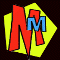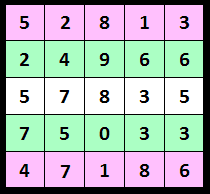#The Massive Number Prediction

## THE SECRET!

There are two parts to this trick.

The first part is writing the prediction.
All you do is look at the digits in the middle row. You write it out, but put "2" on the front and subtract 2 from the end. In this case 57835 becomes 257833.

The second part is forcing the answer. When the top three rows are full, you complete row four. Subtract each digit in row two from 9, and write the answer in row 4. So here, the first digit in row four is 9-2 =7. The next digit is 9-4=5 and so on. When you have completed row four, you complete row five. This time you subract the digits on row one from 9. So here we start with 9-5 =4, then 9-2 =7 and so on.

Once you have completed the grid, get the volunteer to add the numbers and the trick is done!

## Why does it work?The numbers on rows one + five add to 99999.

Also the numbers on rows two + four add to 99999.

If we had added 99999 to the row in the middle, all we needed to do was add 100000 and then subtract 1. But as the trick adds two lots of 99999 to the middle number, it's the same as adding 200000 and then subtracting 2 ... which is what you did when you wrote the prediction out!